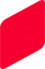# Low-g Accelerometers

This page shows the characteristics of single crystal silicon that measures minute capacitance change and the detection principle.

Basic knowledge of MEMS Technology

##Low-g Accelerometer

The principle of acceleration sensing is simple and reliable: inertia of a moving body is converted into force according to Newton's second law. This is the same phenomenon that gives human beings a sense of acceleration or motion.

The basic elements of the accelerometer are the spring, the proof mass and the surrounding support structures. The spring connects the mass to the support. When the speed of the sensor changes, the proof mass is forced to follow the change via the spring coupling. A force is needed to change the motion of the proof mass. Due to this force the spring is bent and the distance between the body and the proof mass changes in proportion to the acceleration.

In a capacitive sensor the support and the proof mass are insulated from each other, and their capacitance, or charge storage capacity, is measured. As the distance decreases, the capacitance increases and electric current travels towards the sensor; when the distance increases, the opposite occurs. The sensor converts the acceleration of the body into an electric current, charge or voltage.

The superb performance of MEMS sensors based on capacitive measurement technology makes them highly suitable for detecting small changes in motion. The capacitive sensing element measures acceleration in both positive and negative directions and is sensitive to both static acceleration and vibration.

The acceleration sensing elements are made of single crystal silicon and glass. This gives the sensor exceptional reliability, unprecedented accuracy and outstanding stability in terms of time and temperature. Typically our sensing element can withstand more than 50,000 g of acceleration (1 g = the acceleration caused by the Earth's gravity).

The core of our one-axis accelerometer and inclinometer is a symmetrical, bulk-micromachined acceleration sensing element that has two sensing capacitors. Symmetry decreases temperature dependence and cross-axis sensitivity while improving linearity. Hermeticity is achieved by using anodic bonding to attach the wafers to each other. This facilitates the packaging of the element, improves reliability and enables the use of gas damping in the sensor element.

three-axis accelerometer concept offers a single element solution to measure the low-g acceleration vector, three orthogonal components or the magnitude and the direction accurately. The sensor design is based on multiple proof masses suspended asymmetrically with torsion springs. Sensitivity in all three directions is achieved by shifting the centers of gravity of the proof masses from the torsion axes in two directions. Capacitive sensing is used for each proof mass similar to the one-axis sensor. The concept enables very similar linear responses in all three measurement axes.